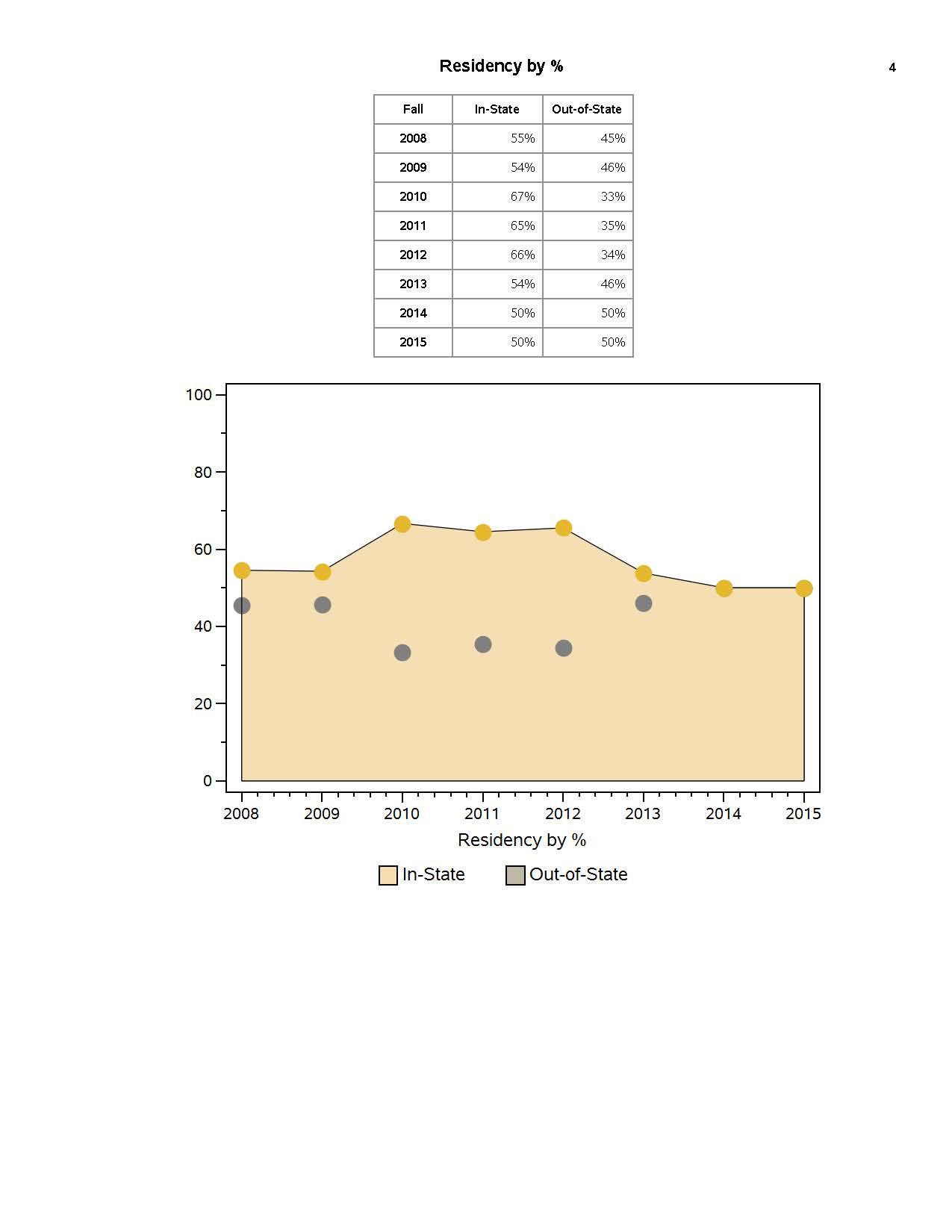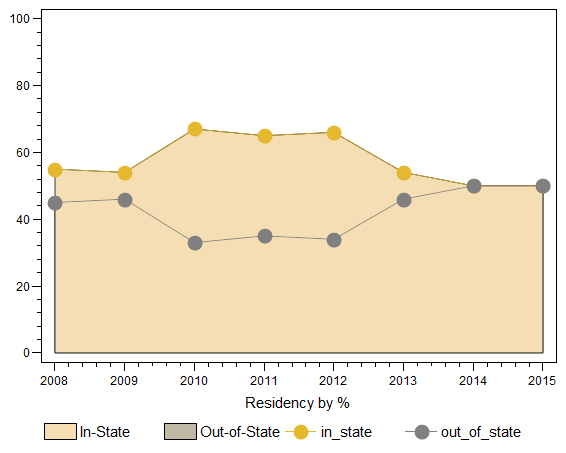## How to show a connecting line in an area-filled overlay plot?

I used proc gplot to produce the graph below.  As the out-of-state area (represented by gray dots) was smaller than the in-state area and was specified as the second layer, the line connecting the gray dots were somehow disappeared.  Do you know how to get the connecting line displayed in this particular case?  Thank you very much.

--------------------------------------------------------------------------------------

Below are the codes I used:

goptions /*reset=all*/ hsize=15cm vsize=12cm;

pattern1 color=wheat;

pattern2 color=brpk;

symbol1 interpol=join value=dot h=1.5 color=bioy;

symbol2 interpol=join value=dot h=1.5 color=gray;

legend1 label=none value=(color=black height=1.5 'In-State' 'Out-of-State');

axis1 offset=(2,2)

label=(height=1.5 'Residency by %')

major=(height=2)

minor=(height=1);

axis2 order=(0 to 100 by 20) offset=(0,0)

label=none

major=(height=2)

minor=(height=1);

title1;

proc gplot data=test4c;

/ line

overlay

haxis=axis1

hminor=4

vaxis=axis2

vminor=1

caxis=black

areas=2

legend=legend1

;run; quit;5 REPLIES 5DanH_sas
SAS Super FREQ

## Re: How to show a connecting line in an area-filled overlay plot?

What happens if you flip the PATTERN definitions, SYMBOL definitions, and the order of the pairs on the PLOT statement?

## Re: How to show a connecting line in an area-filled overlay plot?

Thank you Dan.  I would not want to do it as I wanted to keep it in the same order as the data table above (in-state, and then out-of-state).  (This order is the standard used in my office.)  Is there any way to work around it?  Thank you.DanH_sas
SAS Super FREQ

## Re: How to show a connecting line in an area-filled overlay plot?

I can't run this to make sure you get the same output, but give this a try and see what you get:

proc sgplot data=test4c;

yaxis label="Residency by %";

xaxis display=(nolabel) offsetmin=0 offsetmax=0;

band x=acad_year upper=in_state lower=0 / fillattrs=(color=wheat) transparency=0.5

name="in" legendlabel="In-State";

band x=acad_year upper=out-of-state lower=0 / fillattrs=(color=wheat)  transparency=0.5

name="out" legendlabel="Out-of-State";

series x=x=acad_year y=in_state / markers lineattrs=(color=bioy);

series x=x=acad_year y=out_of_state / markers lineattrs=(color=gray);

keylegend "in" "out";

run;

## Re: How to show a connecting line in an area-filled overlay plot?

Thank you Dan.  It worked!

## Re: How to show a connecting line in an area-filled overlay plot?

Here's 1 way to do it with gplot. Just add an extra overlay of the same 2 things you're plotting, and the'll be on top (the first time plots the areas, and the 2nd time plots the lines)...

data test4c;

datalines;

2008 55 45

2009 54 46

2010 67 33

2011 65 35

2012 66 34

2013 54 46

2014 50 50

2015 50 50

;

run;

goptions /*reset=all*/ hsize=15cm vsize=12cm;

pattern1 color=wheat;

pattern2 color=brpk;

symbol1 interpol=join value=none color=bioy;

symbol2 interpol=join value=none color=gray;

symbol3 interpol=join line=1 value=dot h=1.5 color=bioy;

symbol4 interpol=join line=1 value=dot h=1.5 color=gray;

legend1 label=none value=(color=black height=1.5 'In-State' 'Out-of-State');

axis1 offset=(2,2)

label=(height=1.5 'Residency by %')

major=(height=2)

minor=(height=1 number=4);

axis2 order=(0 to 100 by 20) offset=(0,0)

label=none

major=(height=2)

minor=(height=1 number=4);

title1;

goptions device=actximg;

proc gplot data=test4c;

/ overlay areas=2

haxis=axis1 vaxis=axis2

caxis=black legend=legend1;

run; quit;Discussion stats
• 5 replies
• 973 views
• 6 likes
• 3 in conversation